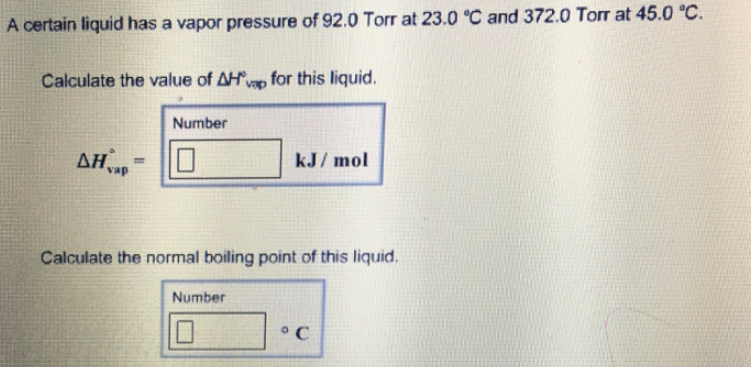# Problem: A certain liquid has a vapor pressure of 92.0 Torr at 23.0°C and 372.0 Torr at 45.0°C.Calculate the value of ΔH°vap for this liquid. Calculate the normal boiling point of this liquid.

###### FREE Expert Solution
93% (156 ratings)###### Problem Details

A certain liquid has a vapor pressure of 92.0 Torr at 23.0°C and 372.0 Torr at 45.0°C.

Calculate the value of ΔH°vap for this liquid.

Calculate the normal boiling point of this liquid.